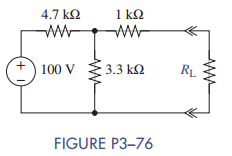### Create an Account

Home / Questions / For the circuit of Figure P3 76 find the value of RL that will result in a Maximum voltage...

# For the circuit of Figure P3 76 find the value of RL that will result in a Maximum voltage. What is that voltage b Maximum current What is that current c Maximum power What is that power

For the circuit of Figure P3−76, find the value of RL that will result in:

(a) Maximum voltage. What is that voltage?

(b) Maximum current. What is that current?

(c) Maximum power. What is that power?Jun 15 2020 View more View LessSubscribe To Get Solution